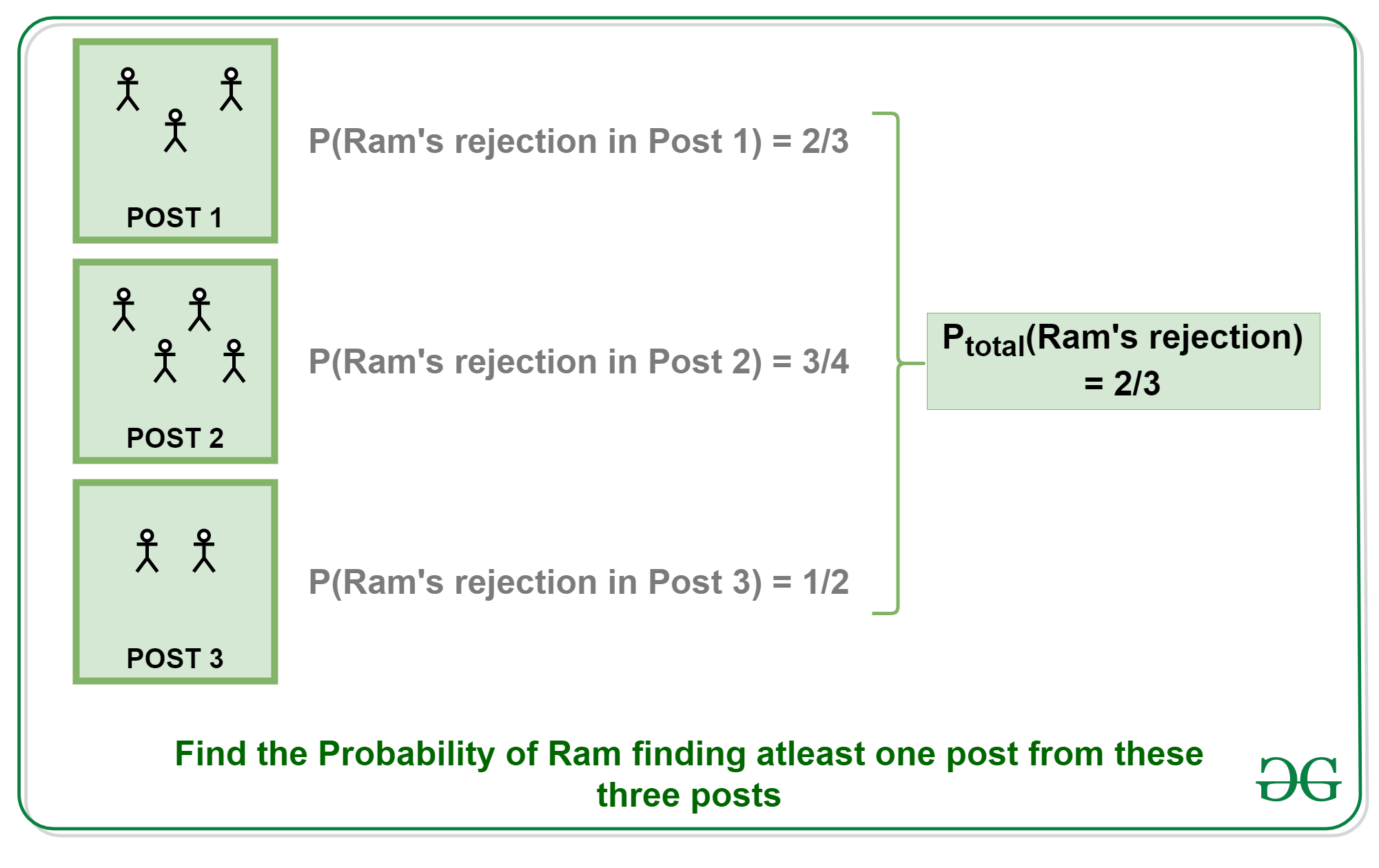Open in App
Not now

# Puzzle | Probability of finding a job

• Difficulty Level : Easy
• Last Updated : 18 Jan, 2023

Puzzle: There is a interview going on and Ram is selected for interview to 3 posts. The total number of candidates appearing for the first, second and the third posts are 3, 4 and 2 respectively. Find the probability of Ram getting at least one job.
Assume that the probabilities are independent of each other.

Solution:

1. Since we need to find the probability of Ram getting at least one job, let’s try to find the probability of Ram not getting any jobs.
2. Probability of Ram getting rejected from the interview of the first post is 2/3.
3. Probability of Ram getting rejected from the interview of the second post is 3/4.
4. Probability of Ram getting rejected from the interview of the third post is 1/2.
5.6. Thus the probability of Ram getting rejected from all of the posts = (2/3)*(3/4)*(1/2) = 6/24 = 1/4.
7. Therefore the probability of Ram getting at least one offer = 1-(1/4) = 3/4.
My Personal Notes arrow_drop_up
Related Articles